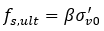# Cohesive

The following properties are required or available for cohesive soil layers:

• Reduction Factors (%) – percent decrease in computed unit resistance for layer
• Skin Resistance Loss
• End Bearing Loss
• Unit Resistance Limits – maximum value of unit resistance
• Skin Friction Limit
• End Bearing Limit

Note that the skin friction resistance is capped by 5% of the entered Concrete Cylinder Strength in the pile properties.

The following parameters are required for each method as described below. For more information see Bored Capacity Theory Manual.

## Total Stress Calculations – Alpha Method

Skin Friction and End Bearing Parameters:

• Undrained Shear Strength, Su

Skin Friction Parameters:

• Alpha (α)

The ultimate unit skin (frictional) resistance (fs,ult) is computed as follows:

fs,ult =α Su

End Bearing Parameters:

• Bearing Capacity Factor Nc

The ultimate unit end (base) resistance (qb,ult) is computed as follows:

qb,ult = Nc Su

## Effective Stress Calculations – Beta Method

Skin Friction Parameters:

• Beta (β)

The ultimate unit skin (frictional) resistance (fs,ult) is computed as follows:Where:End Bearing Parameters:

• Effective Cohesion, c’
• Bearing Capacity Factor Nc
• Bearing Capacity Factor Nq

The ultimate unit end (base) resistance (qb,ult) is computed as follows:Where: